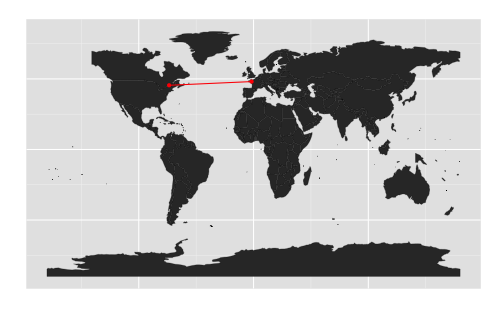We sometimes need the distance between two countries in econometric models, for instance in gravity models. Bur for some countries, I think this measure could cause problem. Let’s take an example. If we want to model the volume of trade between country (i) and country (j), the economic theory says it will depend on the distance between (i) and (j). Let (i) be China, (j_1) Japan, and (j_2) India. The distance between (i) and (j_1) will be lower than between (i) and (j_2). Can we consider, though, that Japan is farther from China than India is?

So, rather than compute the distance between two head cities, it might be more accurate to compute the closest distance between the borders. If a border is shared by country (i) and country (j), then the distance should be zero.

This is extremely simple to do using R, and it gives me an occasion to use the rbind_all function from the R package dplyr. We could obtain the table even faster using cbind_all, but at the moment it is still in development.

[Edit 2017-11_17: See in the comments below and updated and improved version of this by Matt T.]

Note that the relation is symmetric, hence we can optimize the computation.

library(maps)
library(geosphere)
library(dplyr)
world.map <- map("world", fill = TRUE)

indicePays <- seq(1,length(world.map$names))[-grep(":", world.map$names)]

# https://stat.ethz.ch/pipermail/r-help/2010-April/237031.html
splitNA <- function(x){
idx <- 1 + cumsum(is.na(x))
not.na <- !is.na(x)
split(x[not.na], idx[not.na])
}

# Coordinates of every country
lesCoordsX <- splitNA(world.map$x) lesCoordsY <- splitNA(world.map$y)

lesDistancesUnPays <- function(unIndicePays){
# Borders coordinates for current country
coordsPays <- data.frame(long = lesCoordsX[[unIndicePays]], lat = lesCoordsY[[unIndicePays]])

# Indexes of countries except the current one
# and the one for which the computation has already been done
lesIndicesAutresPays <- indicePays[indicePays > unIndicePays]

distancePoint <- function(unPoint){
unPoint.m <- matrix(unPoint, ncol = 2)

# We need to compute distances between unPoint and every border points of every other countries
# it is given by lesIndicesAutresPays

distancePointPays <- function(unIndicePays2){
coordsPays2 <- matrix(cbind(long = lesCoordsX[[unIndicePays2]], lat = lesCoordsY[[unIndicePays2]]), ncol = 2)
lesDistPointPays2 <- spDists(x=coordsPays2, y=matrix(unPoint, ncol=2), longlat=TRUE)
return(min(lesDistPointPays2)) # shortest distance between unPoint and country which index is unIndicePays2
}
lesDistPointPays2 <- lapply(lesIndicesAutresPays, distancePointPays)
res <- unlist(lesDistPointPays2)
return(res)
}

distancesPays <- apply(coordsPays, 1, distancePoint)
# Shortest distances between unPoint and every other country
if(!is.matrix(distancesPays)){
# For the last country on the list
plusCourtesDistances <- min(distancesPays)
}else{
plusCourtesDistances <- apply(distancesPays, 1, min)
}

resul <- cbind(pays1 = rep(unIndicePays, length(plusCourtesDistances)),pays2 = lesIndicesAutresPays, dist = plusCourtesDistances)
return(resul)
}

# We don't need distances for the last country (they have all been computed)
lesDist <- lapply(indicePays[-length(indicePays)], lesDistancesUnPays)
lesDist <- rbind_all(lesDist)

# We need to recover distances for each couple
lesDist$ID <- paste(sprintf("%04d", lesDist$pays1), sprintf("%04d", lesDist$pays2), sep = "") lesDist2 <- data.frame(cbind(pays1 = rep(indicePays, each = length(indicePays)), pays2 = rep(indicePays, length(indicePays)))) lesDist2 <- lesDist2[-which(lesDist2$pays1 == lesDist2$pays2),] lesDist2$ID <- paste(sprintf("%04d", lesDist2$pays1), sprintf("%04d", lesDist2$pays2), sep = "")
lesDist2$ID2 <- paste(sprintf("%04d", lesDist2$pays2), sprintf("%04d", lesDist2$pays1), sep = "") lesDist2$match <- match(lesDist2$ID, lesDist$ID)
lesDist2[is.na(lesDist2$match),"match"] <- match(lesDist2$ID2[is.na(lesDist2$match)], lesDist$ID)
lesDist2$dist <- lesDist[lesDist2$match, "dist"]
lesDist2 <- lesDist2[,c("pays1", "pays2", "dist")]

lesDist <- lesDist2
rm(lesDist2)

# Let's add countries names
lesDist$pays1 <- world.map$names[lesDist$pays1] lesDist$pays2 <- world.map$names[lesDist$pays2]


There you go. If you wish to use these distances, here is the CSV file. You could also download the RData file.

> load(url("http://egallic.fr/R/Blog/Cartes/countries_distances.RData"))
pays1        pays2      dist
1 Canada South Africa 11225.350
2 Canada      Denmark  3963.909
3 Canada         USSR  1254.421
4 Canada     Pakistan  7831.515
5 Canada     Aral Sea  6706.607
6 Canada        Italy  4466.916


## 9 thoughts on “Closest distance between countries”

1.chris says:

what is the unit for this distance?

1.Ewen Gallic says:

It is in kilometres.

2.Hans says:

This was exactly what I needed – sadly the data is ancient. I can’t use distance to USSR when trying to figure out distance from Denmark to a number of former USSR countries 🙁

Really cool work though

1.Ewen Gallic says:

You can easily adapt the code with more recent data though!

3.Matt T says:

Hi Ewen, this is great, exactly what I needed. However, I found that your data excluded island countries such as the UK, New Zealand, Japan, etc. Your script also didn’t work on the latest version of R (3.4.2). I adapted your code to provide for all countries. Code is here: https://gist.githubusercontent.com/mtriff/185e15be85b44547ed110e412a1771bf/raw/1bb4d287f79ca07f63d4c56110099c26e7c6ee7d/getCountryDist.r and CSV output is here: https://gist.githubusercontent.com/mtriff/185e15be85b44547ed110e412a1771bf/raw/1bb4d287f79ca07f63d4c56110099c26e7c6ee7d/countries_distances.csv. Hope this helps someone else.

1.Ewen Gallic says:

Hi Matt,
Thank you!

4.Jess says:

Hi Guys, this is great thank you! Any particular reason why the distance between France and Germany isn’t 0?

1.SrbHrv says:

Many bordering countries have non-zero distance.

I presume it’s because of the inaccuracies in the data set, but can’t be sure.

1.Ewen Gallic says:

Maybe you can define a threshold value? If the distance between bordering countries are are lower than that threshold you can consider that they actually share a border?